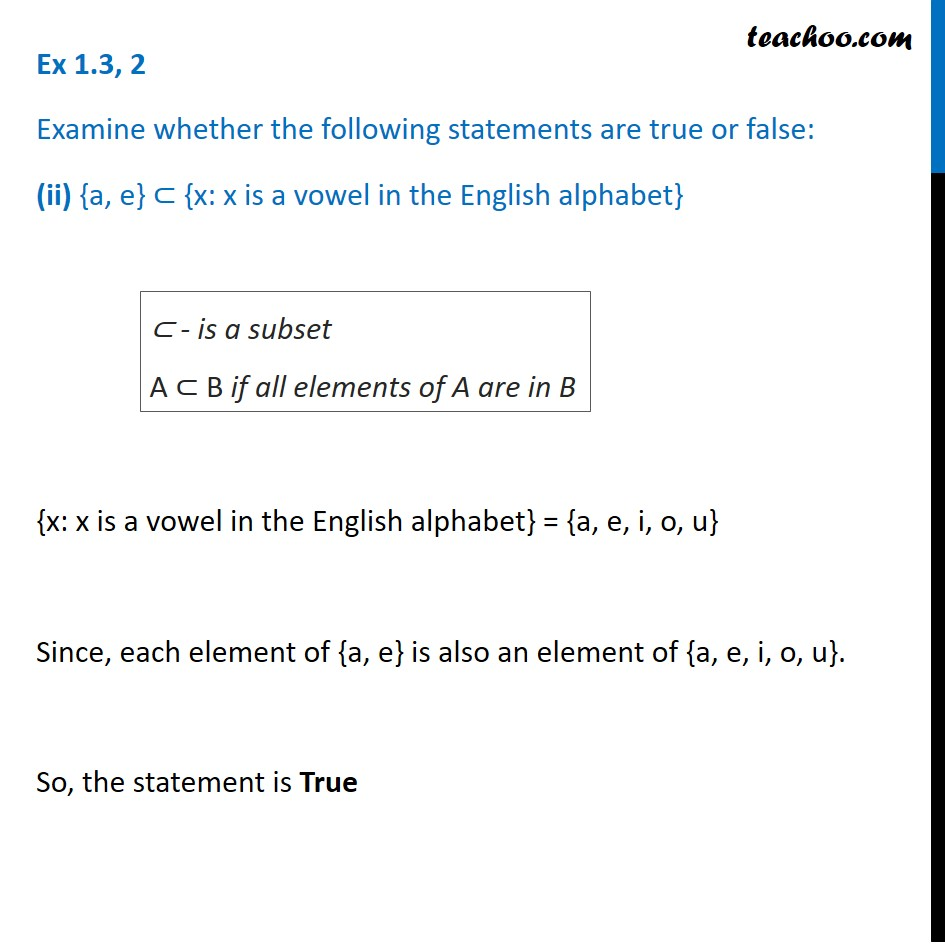1. Chapter 1 Class 11 Sets (Term 1)
2. Serial order wise
3. Ex 1.3

Transcript

Ex 1.3, 2 Examine whether the following statements are true or false: (ii) {a, e} ⊂ {x: x is a vowel in the English alphabet} {x: x is a vowel in the English alphabet} = {a, e, i, o, u} Since, each element of {a, e} is also an element of {a, e, i, o, u}. So, the statement is True ⊂ - is a subset A ⊂ B if all elements of A are in B